# Class 9 RD Sharma Solutions – Chapter 14 Quadrilaterals- Exercise 14.4 | Set 1

### Question 1. In ΔABC, D, E and F are, respectively the mid points of BC, CA and AB. If the lengths of sides AB, BC and CA are 7cm, 8 cm, and 9 cm, respectively, find the perimeter of ΔDEF.

Solution: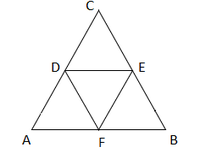From the question it is given that

AB = 7 cm, BC = 8 cm, AC = 9 cm

Find: the perimeter of ΔDEF

In ∆ABC,

D, E and F are the mid points of BC, CA and AB.

So, by midpoint theorem

EF = 1/2 BC,

DF = 1/2 AC and DE = 1/2 AB

Now, we find the perimeter of ΔDEF

So, Perimeter of ∆DEF = DE + EF + DF

= (1/2) AB + (1/2) BC + (1/2) AC

= 1/2 (AB + BC + AC)

= 1/2(7 + 8 + 9)

= 1/2 (24)

= 12 cm

Hence, the perimeter of ΔDEF is 12 cm

### Question 2. In a ΔABC, ∠A = 50°, ∠B = 60° and ∠C = 70°. Find the measures of the angles of the triangle formed by joining the mid-points of the sides of this triangle.

Solution: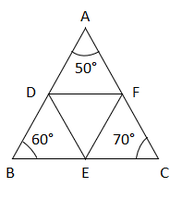From the question it is given that

∠A = 50°, ∠B = 60° and ∠C = 70°

In ΔABC,

D, E, and F are mid points of AB, BC, and AC

So from Midpoint Theorem

DE ∥ AC, DE = (1/2) AC

DE = (1/2) AC = CF

DE ∥ AC, DE = CF [Proved above]

Hence, DECF is a parallelogram.

So, ∠C = ∠D = 70°  [Because the opposite sides of a parallelogram are equal]

Similarly,

BEFD is a parallelogram,

So, ∠B = ∠F = 60°

So, ∠A = ∠E = 50°

Hence, the angles of ΔDEF are

∠D = 70°, ∠E = 50°, ∠F = 60°

### Question 3. In a triangle, P, Q and R are the mid points of sides BC, CA and AB respectively. If AC = 21cm, BC = 29 cm and AB = 30 cm, find the perimeter of the quadrilateral ARPQ.

Solution:From the question it is given that

AC = 21cm, BC = 29 cm and AB = 30 cm

In ΔABC,

R and P are mid points of AB and BC

So from Midpoint Theorem

RP ∥ AC, RP = (1/2) AC

RP ∥ AQ, RP = AQ [Because the opposite sides of a parallelogram

are equal and parallel to each other]

Hence, AQPR is a parallelogram

Now,

AR = (1/2) AB = 1/2 x 30 = 15 cm

So, AR = QP = 15 cm [Because the opposite sides of a parallelogram are equal]

Similarly,

RP = (1/2) AC =1/2 x 21 = 10.5 cm

So, RP = AQ = 10.5 cm [Because the opposite sides of a parallelogram are equal]

Now we find the perimeter of the quadrilateral ARPQ

So, Perimeter of ARPQ = AR + QP + RP + AQ

= 15 + 15 + 10.5 + 10.5

= 51 cm

Hence, the perimeter of the ARPQ = 51cm

### Question 4. In a ΔABC median AD is produced to x such that AD = DX. Prove that ABXC is a parallelogram.

Solution: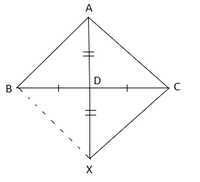From the question it is given that

BD = DC

To prove: Prove that ABXC is a parallelogram.

Proof: Now,

In a quadrilateral ABXC, we have

BD = DC               [Given]

So, diagonals AX and BC bisect each other.

Hence, ABXC is a parallelogram

### Question 5. In a ΔABC, E and F are the mid-points of AC and AB respectively. The altitude AP to BC intersects FE at Q. Prove that AQ = QP.

Solution: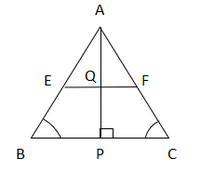In ΔABC

E and F are mid points of AB and AC

So from midpoint theorem

EF ∥ FE, (1/2) BC = FE

Similarly,

In ΔABP

F is the mid-point of AB

So, from mid-point theorem

So, FQ ∥ BP [since EF ∥ BP]

Q is the mid-point of AP

Hence AQ = QP

### Question 6. In a ΔABC, BM and CN are perpendiculars from B and C respectively on any line passing through A. If L is the mid-point of BC, prove that ML = NL.

Solution: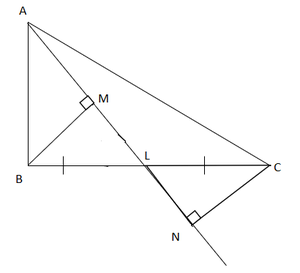In ΔBLM and ΔCLN

∠BML = ∠CNL = 90°

BL = CL         [Because L is the mid-point of BC]

∠MLB = ∠NLC      [Because vertically opposite angle]

So, ΔBLM ΔCLN

Hence, by corresponding parts of congruent triangles

LM = LN

### (ii) The area of ΔADE.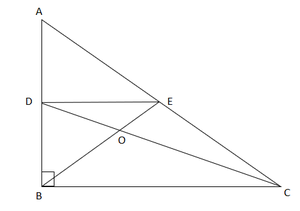Solution:

From the question it is given that

AB = 9 cm, AC = 15 cm, ∠B = 90°

D, E are the mid-points of AB and AC

In ΔABC,

Using Pythagoras theorem

AC2 = AB2 + BC

= 152 = 92 + BC2

= BC2 = 225 – 81 = 144

BC = 12

Similarly,

AD = DB = AB/2 = 9/2 = 4.5 cm     [D is the mid−point of AB]

D and E are mid-points of AB and AC

So, from mid-point theorem

DE ∥ BC ⇒ DE = BC/2

Now, we find the area of ΔADE

So, Area = 1/2 x AD x DE

= 1/2 x 4.5 x 6

= 13.5

Hence, the area of ΔADE is 13.5 cm2

### Question 8. In figure, M, N and P are mid-points of AB, AC and BC respectively. If MN = 3 cm, NP = 3.5 cm and MP = 2.5 cm, calculate BC, AB and AC.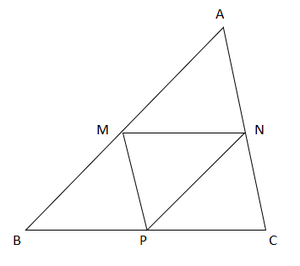Solution:

From the question it is given that

MN = 3 cm, NP = 3.5 cm and MP = 2.5 cm.

Find: the value of BC, AB and AC

In ΔABC

M and N are mid-points of AB and AC

So, from mid-point theorem

MN = (1/2) BC, MN ∥ BC

= 3 = (1/2) BC

= 3 x 2 = BC

= BC = 6 cm

Similarly,

AC = 2MP = 2 (2.5) = 5 cm

AB = 2 NP = 2 (3.5) = 7 cm

Hence, values of BC, AB, and AC are 6 cm,7 cm, and 5 cm

### Question 9. ABC is a triangle and through A, B, C lines are drawn parallel to BC, CA and AB respectively intersecting at P, Q and R. Prove that the perimeter of ΔPQR is double the perimeter of ΔABC.

Solution: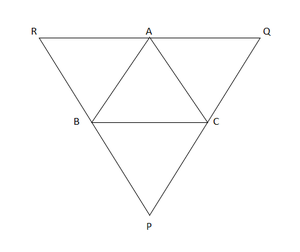To prove: Perimeter of ΔPQR is double the perimeter of ΔABC.

Proof:

In ΔABC

It is given that the ΔABC pass through A, B, C lines are drawn

parallel to BC, CA and AB and intersecting at P, Q and R.

So, ABCQ and ARBC are parallelograms.

BC = AQ and BC = AR          [ Because the opposite sides of a parallelogram are equal]

= AQ = AR

= A is the mid-point of QR

Now we know that,

B and C are the mid points of PR and PQ

So, from mid-point theorem

AB = (1/2) PQ, BC = (1/2) QR, CA = (1/2) PR

= PQ = 2AB, QR = 2BC and PR = 2CA

= PQ + QR + RP = 2 (AB + BC + CA)

Perimeter of ΔPQR = 2 (perimeter of ΔABC)

Hence proved.

### Question 10. In figure, BE⊥ AC, AD is any line from A to BC intersecting BE in H. P, Q and R are respectively the mid-points of AH, AB, and BC. Prove that ∠PQR = 90°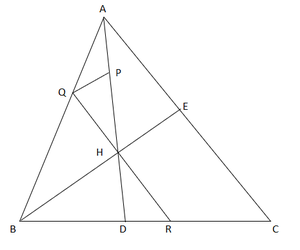Solution:

From the question it is given that

BE ⊥ AC and P, Q and R are the mid-point of AH, AB and BC.

To prove: ∠PQR = 90°

Proof:

In ΔABC,

Q and R are mid-points of AB and BC

So, from mid-point theorem

QR ∥ AC     ….. (i)

In ΔABH,

Q and P are the mid-points of AB and AH

So, from mid-point theorem

QP ∥ BH   ….. (ii)

But, BE⊥AC

So, from eq(i) and (ii) we have,

QP⊥QR

∠PQR = 90°

Hence Proved

Whether you're preparing for your first job interview or aiming to upskill in this ever-evolving tech landscape, GeeksforGeeks Courses are your key to success. We provide top-quality content at affordable prices, all geared towards accelerating your growth in a time-bound manner. Join the millions we've already empowered, and we're here to do the same for you. Don't miss out - check it out now!

Previous
Next